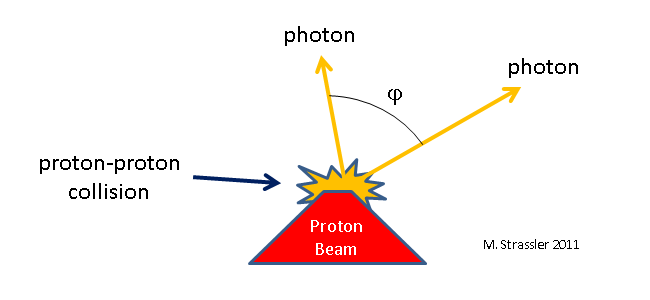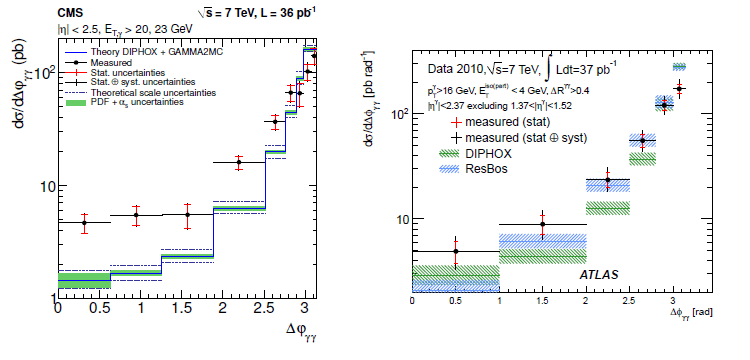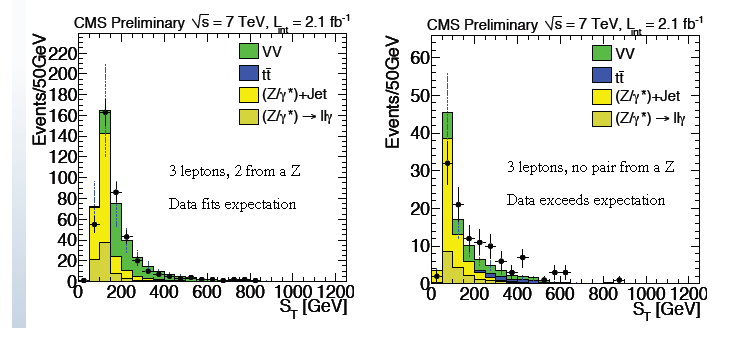# Two-Photons: Data and Theory Disagree

Matt Strassler 11/1/11.   No rest for the weary: yet another discrepancy. This one is somewhat different from the small multi-lepton excess at CMS of a couple of weeks ago (tenuous, but in a very interesting and plausible place) and from the OPERA faster-than-light neutrino claim (not so tenuous, but not so plausible either). Now we have a discrepancy involving collisions that produce two low-energy photons [particles of light]. The effect is seen in four experiments, not one: both ATLAS and CMS at the Large Hadron Collider (LHC), and also CDF and DZero at the Tevatron collider. It’s too large to be a statistical fluke (the excess is not small and it shows up in four experiments). Nor does it look like an experimental mistake (since it shows up in four experiments). Might it be a sign of a new phenomenon not predicted by the Standard Model (the equations that describe the known particles and forces, plus the simplest possible Higgs particle)? Maybe… Can’t rule it out, though there’s not enough information in the experiments’ public documents for a serious evaluation of that possibility. But in any case, my preliminary impression is that it’s most likely something else: either a problem with the theoretical calculation of what the Standard Model predicts, or a problem with the way this theoretical calculation was used by the experiments.

Now why would I come to that conclusion? [and I do emphasize that it is preliminary; I might change my mind, or come to a more focused conclusion, once I obtain more information.] (In what follows, I thank Matt Schwartz, David Krohn, Adam Martin, Andrey Katz and Zhenyu Han for discussing this with me today.)

First let’s look at the data from the LHC. Take the data from 2010 (a small data set, less than 1% of the data taken in 2011), and look at any proton-proton collision that produces two photons which

• are isolated from other particles (for instance, they are not close in angle to any jet [a manifestation of a quark or gluon]) and
• have a certain minimum amount of energy (actually, a minimum amount of momentum perpendicular to the beam.)Fig. 1: In Figures 2 and 3, what is plotted on the horizontal axis is the angle, in the plane perpendicular to the beam direction, between the two photons. Other particles produced in the collision are not shown here.

Now ask: if I look at the directions of motion of the two photons as viewed from the beam — i.e., if I project their motion into the tranverse plane perpendicular to the beam — what is the angle φ between the two photons (see Figure 1)? Plot the number of two-photon events versus that angle. [Actually, first divide that number by the total amount of data, to get a slightly more obscure quantity; this isn’t essential here.] In Figure 2 is what CMS observes, at left, and what ATLAS observes, at right. Because ATLAS and CMS have slightly different requirements on the photons, the plotted data differs quantitatively, but qualitatively the shape of the distribution of events is similar.

Now, CMS states there is a discrepancy between data and theory. And you can see that in the plot. The data are the dots (with error bars shown vertically, and bin-width shown horizontally) while the theoretical prediction is the green area down below the data. For any angle smaller than about 2.8 radians (about 160 degrees) the data exceeds the theory prediction by quite a lot.Fig. 2: The distribution of two-photon events at the LHC, as a function of the angle between the two photons. Left: data from CMS (black dots) and a prediction (green) using a combination of the DIPHOX and GAMMA2MC calculations. Right: data from ATLAS (black dots) and two predictions, one using the DIPHOX calculation (green) and one using the RESBOS calculation (blue). The vertical widths of bars shows estimates of uncertainties; but notice that DIPHOX and RESBOS in the ATLAS plot differ from each other by more than the uncertainty bars for either calculation.

What about ATLAS? Well, here you notice something interesting. The data look quite similar to CMS. But there are two theory predictions. One, in green, lies quite a bit below the data. The other, in blue, is in most regions not at all so far below the data.

Wait a minute. What’s going on here? Isn’t there just one Standard Model? Why are there two predictions here?! In the ATLAS plot, is there a discrepancy between theory and data, or isn’t there?

Ah. Welcome to my world: the world of hadron collider physics. For many measurements at hadron colliders, including the LHC and the Tevatron, calculations of theoretical predictions are not easy. There’s only one Standard Model, but in calculating the prediction of the Standard Model no theorist can do a perfect job — approximations have to be made. Current theoretical methods often do not permit a highly precise calculation, and different people’s calculations often involve different approximations. It is not particularly unusual for theoretical predictions of certain processes at hadron colliders to differ by 50% or more, as you can see in the ATLAS plot. The two predictions shown there are both obtained from reputable theorists who did these tricky calculations, and wrote computer programs with funny names like DIPHOX and RESBOS that make it easier to use the results of their calculations. But they used different approximations, and consequently get somewhat different answers; their methods aren’t wrong, just imprecise, and valid only for certain questions. ATLAS has accounted for this imprecision by citing two theory predictions. They would claim no discrepancy: they would say that theory is rather imprecise here, and the data differs no more from the theoretical predictions than the theoretical predictions differ from each other. CMS, for some reason — maybe a good one, but the reason is not stated in their paper — has instead has chosen only one of the two theoretical predictions shown by ATLAS. They say there is a discrepancy. But note they don’t say anything about this discrepancy being due to a new physical effect not predicted by the Standard Model.  When data deviates from a theoretical prediction, one can only claim observation of a new phenomenon if one has strong confidence in the precision and accuracy of that theoretical prediction.

Let me say right away that I am not an expert in this type of theoretical prediction, and I cannot evaluate for you, at this time, whether one of them is significantly more reliable, or more appropriate for this measurement, than another. Maybe there is a good reason to trust DIPHOX and not RESBOS in this measurement. That being said, I have concerns of my own, after reading the CMS and ATLAS papers and discussing the situation with theory faculty and postdocs at Harvard (where I was a visitor today), as to whether any of the various predictions is correctly implemented by the experimentalists.  I may be able to give you a better picture of the situation once I have collected more information.

By the way, there’s one more thing in Figure 2 that I haven’t mentioned, but perhaps you noticed, that should also make you wonder. For both ATLAS and CMS the number of events at angles close to 3 radians (approaching 180 degrees) is significantly less than predicted by the theoretical calculations.  Since the predictions overshoot here, where the majority of the data is located, one should not be surprised if the entire shape of the distribution comes out wrong.

While we’re at it, the theory predictions also fail badly at CDF and DZero at the Tevatron, for a nearly identical measurement (with vastly more data, but with not that many more events, since the Tevatron ran at lower energy than the LHC). The data is shown in Figure 3, along with different theoretical predictions, all of which fail to a greater or lesser degree. You notice that the nature of the failure is vaguely similar to that shown in the CMS and ATLAS plots, but not exactly the same either.Fig. 3: Similar to Figure 2, but now for the DZero (left) and CDF (right) experiments at the Tevatron collider. Various theoretical calculations are shown in color. Note that the DZero plot covers a smaller region in angle.

Of course you can ask whether the failure of theory to predict this distribution at all four experiments is a signal of a new phenomenon. And there’s nothing here that would exclude that possibility. But my reaction, looking at these plots (and a few others I haven’t shown), is that the theoretical situation is murky, and the observed excesses are most likely due to a problem with the predictions.   Whether that would be due to a failure of the responsible theorists’ calculational technique, or of the way their calculation was implemented by the experimentalists in making the graphs in Figures 2 and 3, is far from clear right now.Fig. 4: The ST distribution (roughly. a measure of the total energy produced in the collision) for events with three leptons at CMS (text inside the plots added by me.) Left: for events where a lepton-antilepton pair are consistent with having arisen from a Z decay, theory and data agree. This suggests theoretical predictions are working well. Right: For events with no such lepton-antilepton pair, data somewhat exceeds theory. The excess is small and not yet significant, but bears watching.

It is interesting to compare this situation with the multi-lepton excess, which, as you may have noticed, I received less skeptically than this two-photon excess. In both cases I know of new physical phenomena that could generate the effect without having been noticed previously.  But there are important differences between the two cases.  On the negative side, the multi-lepton excess seen at CMS has only been seen in one experiment, and is still so small that it might well just be a statistical fluctuation. But on the positive side, there are mild cross-checks of the theoretical predictions for what the Standard Model should give in multi-leptons. Look at the two plots in Figure 4 (taken from CMS’s presentation, with my annotation; see this post.) What they show is that theoretical prediction matches the shape and normalization of the process in the left plot, but fails to do so for the right plot. The left plot serves as a partial cross-check on the prediction that goes into the right plot — by no means a perfect cross-check, but it is reassuring. There are other cross-checks too, made by the experimenters to test the theoretical predictions.  This makes it more plausible to me that the discrepancy in the right plot isn’t due to a problem with the prediction (though that still leaves a statistical fluke, a problem with the measurement, or a new physical phenomenon as possible explanations.)

We don’t, as far as I am aware, have a similar cross-check for the discrepancy seen in Figures 2 and 3 above. [Please correct me if I am wrong!] And that fact makes me suspicious of the theoretical prediction shown in the CMS plot in Figure 2; until independent evidence is shown that it gives correct answers for some other related measurement, I don’t see how we can have much confidence in it.  It would be a mistake, until confidence in the prediction is increased, to draw any strong conclusions about some new phenomenon lurking in this data.

The other interesting difference between the multi-lepton excess and the two-photon excess is that in the former case the experimenters provided us with more information: with the distribution, within the excess events, of three variables: ST, HT and MET. I defined these carefully in the second post on the multi-lepton excess; crudely (and incorrectly), ST measures the overall energy of the event, HT measures the energy carried by jets, and MET is a measure of energy carried off by undetectable particles (such as neutrinos). The fact that the excess in multi-leptons is found at moderate to large ST, and not at small ST, makes it somewhat more plausible that the source of the excess might be a new phenomenon. It would be very helpful to see whether the excess two-photon events in Figure 2 are at large or small ST, and whether there is large HT or MET. If the excess is largest at small ST, HT and MET, it is very unlikely to be from anything new.

Actually, we probably already know there can’t be significant MET (missing transverse momentum, to be precise) in these events. The excess measured by CMS is very large: about 10 pb, or something of order 3000 excess events in the 2010 data. However, there have been searches for events with two photons and MET with 2010 data at ATLAS and CMS. The energy requirements on the two photons are only just slightly more stringent that in the data shown in Figure 2, and the MET distribution is shown for both studies; data fits expectation pretty well. So the photons in Figure 2 cannot be recoiling against something invisible; they also cannot be recoiling against leptons or other photons (something so unusual that it would have been noticed.) Instead, they are probably recoiling against jets.

In the case of the multi-leptons, we were also provided with a plot showing the number of jets per event, which tended to be 0 or 1 in the excess events; see Figure 4 of this post. Such a plot for the two-photon events, which would help distinguish a Standard Model mis-calculation from a new phenomenon, has not been shown for the two photon events. Ordinary Standard Model events with two photons would tend to give 0, 1 or 2 jets. But any new physics source for the two-photon excess, given its high rate, would be hard pressed to give only 2 such jets as a typical number; if indeed the MET in these events is always small, then the number of jets would typically be 4 or more. [To wit: it is hard to make a new heavy particle that is produced in pairs with a high rate and decays to one jet, a low-energy photon, and no MET. There is a way around this though… isn’t there always?… but generally it would show up in the invariant mass of the most energetic two jets.  Sorry that this is cryptic; long story.]

I should add that the experimenters have provided some other plots regarding the two photons (the invariant mass of the pair, the transverse momentum of the pair, some angular information, etc.) Since there isn’t any one plot which seems to me particularly definitive regarding the source of the excess, I’ve decided not to show them here, to keep the discussion here from getting even longer than it already is.

### 4 thoughts on “Two-Photons: Data and Theory Disagree”

1.How come it’s not possible to get correctness bounds on the theoretical prediction? Are they also hard to compute?

•In general, it is almost as hard to estimate the size of the error on one’s approximation as it would be to improve the approximation directly.

One reason is that theoretical calculations of this type tend to involve the computation of various quantities which partially cancel. A close analogy: suppose I wanted to calculate a function f(x) for x less than 1 (but not too much less than 1), but I only knew how to calculate it as a series expansion: f(x) = 3 + 2x + order(x^2). A better approximation would be obtained if I could calculate the next term: f(x) = 3 + 2 x + c x^2 + order(x^3) — which would require me to calculate c. Alternatively, if I want to know how accurate my original approximation of 3 + 2x is, I just need to *estimate* c. But if it turns out that my calculation of c is of the form c = c1 + c2 + c3 + c4 + c5 where c1 = 10 and c2 = -17 and c3 = +12 and c4 = -22 and c5 = 15, so that c = -2, I would not get a very good estimate if I tried to take a short cut and just calculate c1 alone. In such a situation, the size of c — which determines how good my original approximation is — is very difficult to guess; with all those cancellations, it could easily have turned out to be +6 or -0.3 instead of -2, which would make the approximation either much worse or much better than it actually turns out to be.

2.